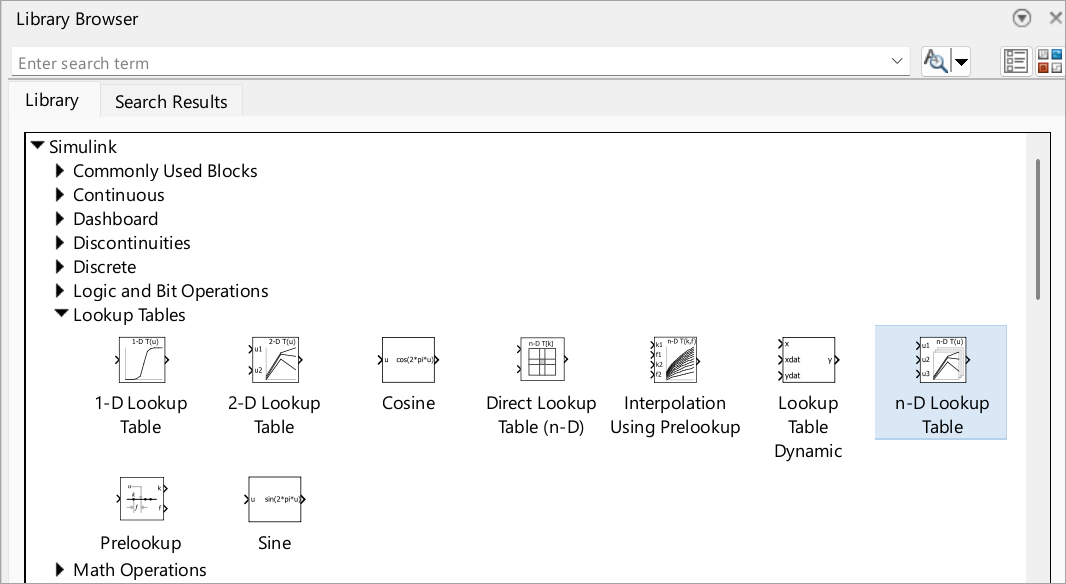Documentation

### This is machine translation

Mouseover text to see original. Click the button below to return to the English version of the page.

# Nonlinearity

Model nonlinearity with lookup tables, approximate mathematical functions by mapping input values to output values

If you are new to modeling nonlinearity, first try using a lookup table block. Lookup table blocks use arrays of data to map input values to output values, approximating mathematical functions. To approximate a function in N variables, use the n-D Lookup Table block:## Blocks

 1-D Lookup Table Approximate one-dimensional function 2-D Lookup Table Approximate two-dimensional function Direct Lookup Table (n-D) Index into n-dimensional table to retrieve element, vector, or 2-D matrix Interpolation Using Prelookup Use precalculated index and fraction values to accelerate approximation of N-dimensional function Lookup Table Dynamic Approximate a one-dimensional function using dynamic table n-D Lookup Table Approximate n-dimensional function Prelookup Compute index and fraction for Interpolation Using Prelookup block Sine, Cosine Implement fixed-point sine or cosine wave using lookup table approach that exploits quarter wave symmetry

## Classes

 `Simulink.LookupTable` Store and share lookup table and breakpoint data, configure the data for ASAP2 and AUTOSAR code generation `Simulink.Breakpoint` Store and share data for a breakpoint set, configure the data for ASAP2 and AUTOSAR code generation `Simulink.lookuptable.Evenspacing` Configure even spacing set data for lookup table object `Simulink.lookuptable.Table` Configure table data for lookup table object `Simulink.lookuptable.Breakpoint` Configure breakpoint set data for lookup table object `Simulink.lookuptable.StructTypeInfo` Configure settings for structure type that lookup table object uses in the generated code

## Examples and How To

Enter Breakpoints and Table Data

How to specify breakpoint data sets and table data for lookup table blocks

Edit Lookup Tables

How to change the elements of lookup tables

Import Lookup Table Data from MATLAB

You can import table and breakpoint data from variables in the MATLAB workspace by referencing them in the Table and Breakpoints tab of the dialog box.

Import Lookup Table Data from Excel

This example shows how to use the MATLAB `xlsread` function in a Simulink model to import data into a lookup table.

Create a Logarithm Lookup Table

Example demonstrating how to work with lookup table blocks

Prelookup and Interpolation Blocks

Provides examples of using Prelookup and Interpolation Using Prelookup blocks together

Update Lookup Table Blocks to New Versions

Process for updating existing models to use current versions of lookup table blocks

Optimize Generated Code for Lookup Table Blocks

Provides tips for optimizing generated code for lookup table blocks

## Concepts

Brief overview of lookup table blocks

Anatomy of a Lookup Table

Introduces the components of a lookup table

Lookup Tables Block Library

Describes the various blocks in the Lookup Tables library

Guidelines for Choosing a Lookup Table

How to select the best lookup table for your application

Characteristics of Lookup Table Data

Describes requirements of lookup table data and outlines how to represent discontinuous data

Methods for Approximating Function Values

How to set the method by which lookup table blocks approximate function values

Lookup Table Glossary

Terminology used to describe lookup tables in Simulink® models